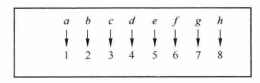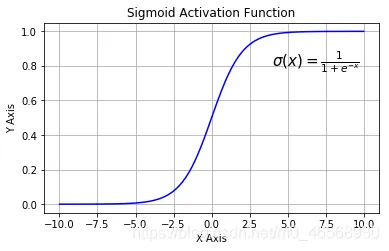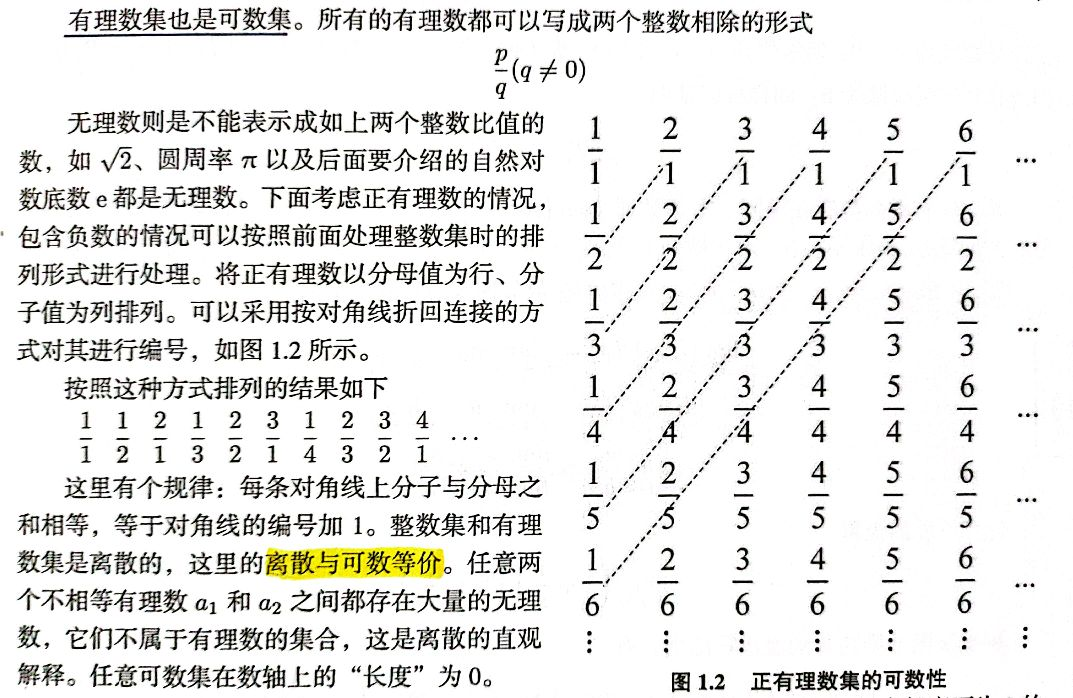# 可数集与不可数集

• 本节的重点在于理解可数与不可数的概念，它们将用于定积分中函数的可积性，以及概率论中的离散型与连续型随机变量等重要概念中。
• 可数集是在“集合等势”概念的基础上进行定义的，因此要理解可数与不可数首先要理解什么是集合等势。
• 本节的讨论范围是“有限集+无限集”，而初等数学的主要讨论对象是有限集。
• 当我们面对“无穷”问题时，必须建立以下几个基本观点：1)有限到无限是从量变到质变；2)有限集的性质不能推广到无限，反之亦然；3)要依靠理性的论证，而不是直观和常识来认识无限。
• 因此首先要放弃以前初等数学中（有限）集合的知识，重现建立“有限集+无限集”下集合的严格定义。

## 一、初等数学中对有限集/无限集及其基数的理解

1. 对有限集和无限集的理解仅仅停留在“直观理解”层面：元素个数有限的集合是有限集；元素个数无限的集合是无限集。
2. 用集合中元素的个数（基数）作为集合大小的度量方式，比如集合$$A=\{a,b,c,d,e,f,g,h\}$$中有8个元素，因此集合$$A$$的基数就是8，记为$$|A|=8$$
3. 可以将集合的基数看作数值直接进行比较，比如集合$$|A|=80$$$$|B|=100$$，因为 $$80 < 100$$，所以 $$|A| < |B|$$
4. 定义了集合间的基本关系（子集、真子集、相等）以及集合的基本运算（交集、并集、补集）。
5. 经常利用集合间关系和维恩图的直观理解进行集合基数的相关运算，比如：
• $|A \cup B| = |A| + |B| – |A \cap B|$
• 如果$$A \subset B$$，则$$|A|<|B|$$
• 如果$$A \cap B = \emptyset$$，则$|A|+|B|=|A \cup B|$

1. 对无限集讨论元素个数是没有意义的，因为所有无限集元素的个数都是$$+\infty$$
2. 某些在有限集下成立的性质在无限集中不再成立，比如“如果$$A \subset B$$，则$$|A|<|B|$$”这条性质就不在成立，举例说明如下：

【例1】对下面几个集合的基数进行比较：

1. 正整数集 $$\mathbb{N^+}$$
2. 正奇数集 $$A_1$$
3. 正偶数集 $$A_2$$

对于【例1】中提到的这三个集合，有下面几组集合关系：

$\mathbb{N^+} = A_1 \cup A_2 \\ A_1 \subset \mathbb{N^+} \\ A_2 \subset \mathbb{N^+} \\ A_1 \cap A_2 = \emptyset$

这是否意味着 $$|A_1| <|\mathbb{N^+}|$$$$|A_2| <|\mathbb{N^+}|$$$$|A_1|+|A_2|=|\mathbb{N^+}|$$ 呢？答案是否定的（实际上这三个集合是等势的）。

## 二、基数的本质

2. 用集合中元素的个数（基数）作为集合大小的度量方式，比如集合$$A=\{a,b,c,d,e,f,g,h\}$$中有8个元素，因此集合$$A$$的基数就是8，记为$$|A|=8$$$我们来深入思考$$|A|=8$$这一简单结论背后的思想： • 判断一个有限集合中元素的“多少”，其实是采用“数数”的方法。比如在集合$$A$$中：a是第一个元素、b是第二个元素、…、h是第8个元素；一个一个去数集合中的元素，等到把所有的元素都数完了（因为是有限集所有才有可能数完），得出该集合中一共有8个元素的结论。 • “数数”的过程其实就是与有限集合$$N_n=\{1,2,…,n\}$$建立“一一对应”的映射关系的过程。比如计算集合$$A$$中元素个数的过程实际上就是建立如下图所示的映射关系的过程：注：在集合基数及其比较.ppt中对“基数的本质”有一些很有意思的讨论，可以了解一下。 ## 三、有限集和无限集的定义 一个集合“能够与有限集合$$N_n=\{1,2,…,n\}$$建立一一对应的映射关系”实际上就是说：如果一个一个数，该集合中的元素是可以数完的。在这样的考虑下可以重新对有限集和无限集进行定义： 【定义1】 一个集合$$S$$与集合$$N_n=\{1,2,…,n\}$$（定义$$N_0 = \emptyset$$）之间如果存在一一对应函数 $$f: S \rightarrow N_n$$，则称$$S$$是有限集，否则称$$S$$是无限集。 【定义2】有限集$$S$$的元素的个数称为S的基数，记为$$|S|$$ 例如，下列集合均为有限集：注意 • 无限集是指“不能与集合$$N_n$$建立一一对应关系的集合”，而不是“能与$$N_n$$建立一一对应关系，但n为无穷大的集合（此时$$N_n$$其实就是正整数集$\mathbb{N^+} $）（这其实是可数集的定义）”。 • “能与$$N_n$$建立一一对应关系”意味着可以一个一个数，而有的无限集比如实数集是根本就不能计数的。 ## 四、 集合等势 假设集合$$A=\{a,b,c,d,e,f,g,h\}, B=\{1月, 2月, 3月, … , 8月\}$$，很容易可以得出“集合$$A$$和集合$$B$$的基数相等”的结论，下面思考这一结论是如何得出的？ • 集合$$A=\{a,b,c,d,e,f,g,h\}$$可以与有限集合$$N_8 = \{1, 2, 3, …, 8\}$$建立一一映射关系 => 集合$$A$$中有8个元素，$$|A|=8$$ • 集合$$B=\{1月, 2月, 3月, … , 8月\}$$可以与有限集合$$N_8 = \{1, 2, 3, …, 8\}$$建立一一映射关系 => 集合$$B$$中有8个元素，$$|B|=8$$ • 因为$$|A|=|B|=8$$，所以集合$$A$$和集合$$B$$的基数相等 从表面上看，得出“集合$$A$$和集合$$B$$的基数相等”这一结论的原因似乎是$$|A|=|B|=8$$，本质上却是“集合$$A$$和集合$$B$$都能与有限集合$$N_8 = \{1, 2, 3, …, 8\}$$建立一一映射关系”，进一步说实际上是“集合$$A$$和集合$$B$$能够建立一一映射关系”。 在这样的考虑下给出两个集合等势的定义： 【定义2】对于集合$$A$$和集合$$B$$，如果集合$$A$$中的任意元素$$a$$，在集合$$B$$中都有唯一的元素$$b$$通过某种映射关系与之对应，即存在如下的从$$A$$$$B$$的双射函数（Bijection，一对一映射函数） $b=f(a), a \in A, b \in B, f: A \rightarrow B$ 则称集合$$A$$与集合$$B$$等势，记为$$A \sim B$$ • 集合的势是一个用来度量集合所含元素多少的量。集合的势越大，所含的元素越多。 • 两个集合等势的定义中并没有对集合的类型进行限制，也就是说上面的定义对有限集和无限集都适用 • 有限集可以直击计算出元素个数（基数），一般不用“势”来度量元素的个数 在【例1】中曾经提到过“正整数集 $$\mathbb{N^+}$$、正奇数集 $$A_1$$和正偶数集 $$A_2$$两两之间等势”，下面对这一结论进行说明： 1. 正整数集 $$\mathbb{N^+}$$与正偶数集 $$A_2$$等势： • 对于集合$$\mathbb{N^+}$$中的每一个元素$$i$$，都有$$A_2$$中的元素$$2i$$与之对应；反过来$$A_2$$中的元素$$i$$也都有 $$\mathbb{N^+}$$中的元素$$\frac{i}{2}$$与之对应。 • 即存在从集合$$\mathbb{N^+}$$到集合$$A_2$$的双射关系：$$i \rightarrow 2i, i \in \mathbb{N^+}, 2i \in A_2$$ • 因此，正整数集 $$\mathbb{N^+}$$与正偶数集 $$A_2$$等势 同理： 1. 因为存在从集合$$\mathbb{N^+}$$到集合$$A_1$$的双射关系：$$i \rightarrow 2i-1, i \in \mathbb{N^+}, 2i-1 \in A_1$$，所以正整数集 $$\mathbb{N^+}$$与正奇数集 $$A_1$$等势； 2. 因为存在从集合$$A_1$$到集合$$A_2$$的双射关系：$$i \rightarrow i+1, i \in A_1, i+1 \in A_2$$，所以正奇数集 $$A_1$$与正偶数集 $$A_2$$等势。 再举一个连续集合的例子：实数集 $$\mathbb{R}$$与区间 $$(0, 1)$$等势 • 因为存在从实数集 $$\mathbb{R}$$到区间 $$(0, 1)$$的双射函数：$$f(x) = \frac{1}{1+e^{-x}}, x \in \mathbb{R}$$，所以实数集 $$\mathbb{R}$$与区间 $$(0, 1)$$等势 • 这一函数也称为logistic函数或sigmoid函数，其函数图像如下图所示：## 五、可数集与不可数集 【定义3】如果集合$$S$$能与正整数集 $$\mathbb{N^+}$$建立一一对应的映射关系，即存在从正整数集 $$\mathbb{N^+}$$到集合$$S$$的双射关系：$$f: \mathbb{N^+} \rightarrow S$$，则称集合$$S$$是可数的。换句话说，与正整数集 $$\mathbb{N^+}$$等势的集合称为可数集。 ### 5.2 对“可数”的理解 因为一开始对“可数”这一概念实在是不能理解，所以查阅了很多资料，先将其中一部分我认为有价值的列出来，这些资料分别从不同角度对“可数”的概念进行了解释： 1. 百度百科： 可数集（Countable set），是指每个元素都能与自然数集N的每个元素之间能建立一一对应的集合。如果将可数集的每个元素标上与它对应的那个自然数记号，那么可数集的元素就可以按自然数的顺序排成一个无穷序列 a1，a2，a3，…an，…。 以下是判断一个集合是可数集合的一些结论。 • 按照可数集合的定义，若A为有限集，则A一定是可数集合，否则若A与自然数集之间存在一个一一对应的映射，则A为可数集合。 • 若A与某可数集合之间存在一一对应的映射，则A为可数集合。 • 若A中所有元素可按某种规律进行排序，则A是可数集合。 • 若A是n(>1)个可数集合的并集，则A是可数集合。 • 若A是某个已知是可数集合的子集，则A是可数集合。 • 若A是n(>1)个可数集合的笛卡儿乘积，则A是可数集合。 1. 维基百科： 在数学上，可数集，或称可列集，是与自然数集的某个子集具有相同基数（等势）的集合。在这个意义下，可数集由有限可数集无限可数集组成。不是可数集的无穷集称为不可数集。这个术语是康托尔创造的。可数集的元素，正如其名，是“可以计数”的：尽管计数有可能永远无法终止，集合中每一个特定的元素都将对应一个自然数。 “可数集”这个术语有时仅仅指代无限可数集，即仅代表能和自然数集本身一一对应的集合。两个定义的差别在于有限集合在前者中算作可数集，而在后者中不算作可数集。为了避免歧义，前一种意义上的可数有时称为至多可数，后一种可数集则称为无限可数集。 …它无非是将我们数数（shǔ shù）的行为进行了数学定义。因为日常，当我们数数的时候，就是沿着自然数的元素一个个数一些对象的个数的… 问：可列集是不是能全列出来就算可列集？ 答：不是说能全列出来，理论上的意思是你对于一个可列集中的元素你总能在排序中确定一个他的位置，或者说这个集合与正整数集存在一一对应关系才叫可列，全列出来是不可能的，因为毕竟是无限集，不过可以说能够一直列下去。 无限集中，所有的可数集都是等势的，其中可数等价于可列，即所有元素可以排成一个无穷数列{a_i}，其中对于集合中的每一个元素，总存在唯一一个自然数下标n，使得a_n就是这个元素。 总结： 其实对“可数”最好的解释就是“可以一个一个地数”或者“可以计数” 本文在「2.1基数的本质」中已经对“计数”这一行为进行了讨论，下面通过对什么是“不可计数”进行说明，以便更好地理解： 【例2】实数集$$\mathbb{R}$$或长度不为0的实数区间是不可数的。 • 这些集合中的元素是连续的，它们在数轴上是相当稠密的。 • 对于长度不为0的实数区间$$(a_1,a_2)$$，在该区间内任意两个不相等的实数（不论它们之间的距离有多近）之间都有无数个实数，它们之间的这些实数同样也属于$$(a_1,a_2)$$实数区间。 • 考虑一个实数区间$ [1, 10 ]\$，试图对它所包含的实数进行计数。一开始先间隔1取数进行计数，数了10个数：1到10；这时发现刚刚取到的10个数中相邻两个数之间仍有无数个数，于是先试着数一数1和2之间的数，这次间隔0.1取数，取到了1.0、1.1、…、1.9、2.0这11个数；然后发现1.1和1.2之间还有无数个数，继续用更小的间隔0.01取数：1.00、1.01、…、1.09、1.10；…这样的过程似乎可以无止尽地进行下去，即使数到了1.0000000000000000001和1.0000000000000000002，它们之间还是有无穷多个数。最后发现根本没有办法对它进行计数。
• “无法计数”并不是说“数不完”，“数不完”的含义其实是“可以数”但是“计数的过程会无止境地进行下去”，“数不完”说的是无限可数集的情况，比如对于正偶数集，我可以按照2、4、6、8、10、…的规律一直往下数但是永远也数不完。

• 离散与可数是等价的

### 5.5 一些可数集的例子

1. 自然数集$$\mathbb{N}$$
2. 整数集$$\mathbb{Z}$$
对于所有的整数$$… ,-4, -3, -2, -1, 0, 1, 2, 3, 4, …$$可以按照下面的顺序一直数下去：$$0, 1,-1,2,-2,3,-3,4,-4,…$$
3. 有理数集$$\mathbb{Q}$$